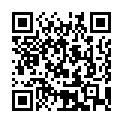# North Coast Synthesis Ltd.

## B♭m4+2+♯1Choose audio sample set:
MSK 007 Leapfrog VCF (patch; see in online store)
MSK 009 Coiler VCF (patch; see in online store)

Equivalent chord symbols: B♭m4+2+♭2, B♭m4+2+♭9, B3+2+♯1+♯7, B3+2+♯1+♭1, D♭2+6+7+♯7, D♭2+6+7+♭1.

Notes in this chord: B♭, B, C, D♭, E♭. Integer notation: {0, 1, 3, 10, 11}.

Nearby chords (one less note): B♭m4+2, B3+2+♯1, D♭2+6+7, B3+♯1+♯7, B♭2+♯1+♯2.

Nearby chords (one more note): BM9+♯1, BM9♭5+♯1, E♭7+6+♯5, A♭+2+4+♯2, B♭4+2+♯1+♯2, Adim+2+♯1+♯3, B♭dim+2+4+♯1.

Parallel chords (same structure, different root): Cm4+2+♯1, Dm4+2+♯1, Em4+2+♯1, Fm4+2+♯1, Gm4+2+♯1, Am4+2+♯1, Bm4+2+♯1, D♭m4+2+♯1, E♭m4+2+♯1, G♭m4+2+♯1, A♭m4+2+♯1.

Experimental fretting charts for guitar standard EADGBE tuning (change tuning or instrument):